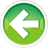# 4.9 — Boolean values

In real-life, it’s common to ask or be asked questions that can be answered with “yes” or “no”. “Is an apple a fruit?” Yes. “Do you like asparagus?” No.

Now consider a similar statement that can be answered with a “true” or “false”: “Apples are a fruit”. It’s clearly true. Or how about, “I like asparagus”. Absolutely false (yuck!).

These kinds of sentences that have only two possible outcomes: yes/true, or no/false are so common, that many programming languages include a special type for dealing with them. That type is called a Boolean type (note: Boolean is properly capitalized in the English language because it’s named after its inventor, George Boole).

Boolean variables

Boolean variables are variables that can have only two possible values: true, and false.

To declare a Boolean variable, we use the keyword bool.

To initialize or assign a true or false value to a Boolean variable, we use the keywords true and false.

Just as the unary minus operator (-) can be used to make an integer negative, the logical NOT operator (!) can be used to flip a Boolean value from true to false, or false to true:

Boolean values are not actually stored in Boolean variables as the words “true” or “false”. Instead, they are stored as integers: true becomes the integer 1, and false becomes the integer 0. Similarly, when Boolean values are evaluated, they don’t actually evaluate to “true” or “false”. They evaluate to the integers 0 (false) or 1 (true). Because Booleans actually store integers, they are considered an integral type.

Printing Boolean variables

When we print Boolean values with std::cout, std::cout prints 0 for false, and 1 for true:

Outputs:

```1
0
0
1
```

If you want std::cout to print “true” or “false” instead of 0 or 1, you can use std::boolalpha:

This prints:

```1
0
true
false
```

You can use std::noboolalpha to turn it back off.

Integer to Boolean conversion

You can’t initialize a Boolean with an integer using uniform initialization:

However, in any context where an integer can be converted to a Boolean , the integer 0 is converted to false, and any other integer is converted to true.

This prints:

```true
false
```

Inputting Boolean values

Inputting Boolean values using std::cin sometimes trips new programmers up.

Consider the following program:

```Enter a Boolean value: true
You entered: 119
```

Wait, what?

It turns out that std::cin only accepts two inputs for Boolean variables: 0 and 1 (not true or false). Any other inputs will cause std::cin to silently fail. In this case, because we entered true, std::cin silently failed and didn’t assign a value to variable b. Consequently, when std::cout printed a value for b, it printed whatever uninitialized value was in variable b. Garbage!

Boolean return values

Boolean values are often used as the return values for functions that check whether something is true or not. Such functions are typically named starting with the word is (e.g. isEqual) or has (e.g. hasCommonDivisor).

Consider the following example, which is quite similar to the above:

Here’s output from two runs of this program:

```Enter an integer: 5
Enter another integer: 5
5 and 5 are equal? true
```
```Enter an integer: 6
Enter another integer: 4
6 and 4 are equal? false
```

How does this work? First we read in integer values for x and y. Next, the expression “isEqual(x, y)” is evaluated. In the first run, this results in a function call to isEqual(5, 5). Inside that function, 5 == 5 is evaluated, producing the value true. The value true is returned back to the caller to be printed by std::cout. In the second run, the call to isEqual(6, 4) returns the value false.

Boolean values take a little bit of getting used to, but once you get your mind wrapped around them, they’re quite refreshing in their simplicity! Boolean values are also a huge part of the language -- you’ll end up using them more than all the other fundamental types put together!

We’ll continue our exploration of Boolean values in the next lesson.4.10 -- Introduction to if statementsIndex4.8 -- Floating point numbers

### 101 comments to 4.9 — Boolean values

•Gayan

#include <iostream>

bool isPrime(int x)
{

return (x==2||x==3||x==5||x==7);
}

int main()
{
using namespace std;
cout<<"Enter a single digit integer! "<<endl;
int x=0;
cin>>x;

bool Equal=isPrime(x);

if(Equal)
cout<<x<<" is a Prime number ! "<<endl;
else
cout<<x<<" is not a Prime number ! "<<endl;

return 0;
}